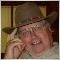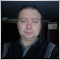Indicator MT-4 to MT-5588

I have coded this Damiani_volatmeter.mq4 MT-4 indicator to MT-5. The output results on EURUSD M15 chart is not the same. Can someone advise why?

MT-4 version

#property indicator_separate_window
#property indicator_buffers 3
#property indicator_color1 Silver
#property indicator_color2 FireBrick
#property indicator_color3 Lime
//---- input parameters
extern int       Viscosity=7;
extern int       Sedimentation=50;
extern double    Threshold_level=1.1;
extern bool      lag_supressor=true;
extern double    lag_s_K=0.5;
//---- buffers
double thresholdBuffer[];
double vol_m[];
double vol_t[];
double ind_c[];
//+------------------------------------------------------------------+
//| Custom indicator initialization function                         |
//+------------------------------------------------------------------+
int init()
{
//---- indicators
SetIndexStyle(0,DRAW_LINE);
SetIndexBuffer(0,thresholdBuffer);
SetIndexStyle(1,DRAW_SECTION);
SetIndexBuffer(1,vol_m);
SetIndexStyle(2,DRAW_LINE);
SetIndexBuffer(2,vol_t);

ArrayResize(ind_c,Bars);
ArrayInitialize(ind_c,0.0);
//----
return(0);
}
//+------------------------------------------------------------------+
//| Custor indicator deinitialization function                       |
//+------------------------------------------------------------------+
int deinit()
{
//----

//----
return(0);
}
//+------------------------------------------------------------------+
//| Custom indicator iteration function                              |
//+------------------------------------------------------------------+
int start()
{
double vol=0;
int    changed_bars=IndicatorCounted();
//Comment("ATR ratio= "+short_atr+" / "+long_atr);
int limit=Bars-changed_bars;
if (limit>Sedimentation+5)limit=limit-Sedimentation;
for(int i=limit;i>=0;i--)
{

double sa=iATR(NULL,0,Viscosity,i);
double s1=ind_c[i+1];
double s3=ind_c[i+3];
double atr=NormalizeDouble(sa,Digits);
if(lag_supressor)
vol= sa/iATR(NULL,0,Sedimentation,i)+lag_s_K*(s1-s3);
else
vol= sa/iATR(NULL,0,Sedimentation,i);
//vol_m[i]=vol;

double anti_thres=iStdDev(NULL,0,Viscosity,0,MODE_LWMA,PRICE_TYPICAL,i);

anti_thres=anti_thres/
iStdDev(NULL,0,Sedimentation,0,MODE_LWMA,PRICE_TYPICAL,i);

double t=Threshold_level;
t=t-anti_thres;

if (vol>t){vol_t[i]=vol;vol_m[i]=vol;
IndicatorShortName("DAMIANI Signal/Noise: TRADE  /  ATR= "+DoubleToStr(atr,Digits)+"    values:");}
else {vol_t[i]=vol;vol_m[i]=EMPTY_VALUE;
IndicatorShortName("DAMIANI Signal/Noise: DO NOT trade  /  ATR= "+DoubleToStr(atr,Digits)+"    values:");}
ind_c[i]=vol;
thresholdBuffer[i]=t;
}
//----

//----
return(0);
}
//+------------------------------------------------------------------+

MT-5 version

#property indicator_separate_window

#property indicator_buffers 8
#property indicator_plots   3

#property indicator_color1 Silver
#property indicator_type1   DRAW_LINE
#property indicator_label1  "Signal"
#property indicator_color2 FireBrick
#property indicator_type2   DRAW_SECTION
#property indicator_label2  "Main"
#property indicator_color3 Lime
#property indicator_type3   DRAW_LINE
#property indicator_label3  "No Trade"

//---- input parameters
input int       Viscosity=7;
input int       Sedimentation=50;
input double    Threshold_level=1.1;
input bool      lag_supressor=true;
input double    lag_s_K=0.5;
//---- buffers
double thresholdBuffer[];
double vol_m[];
double vol_t[];
double ind_c[];
int myATR1, myATR2;
int mySteDev1, mySteDev2;
double ATRBuffer1[];
double ATRBuffer2[];
double SteDevBuffer1[];
double SteDevBuffer2[];

//+------------------------------------------------------------------+
//| Custom indicator initialization function                         |
//+------------------------------------------------------------------+
int OnInit()
{
//--- indicator buffers mapping

//---- indicator line
SetIndexBuffer(0,thresholdBuffer,INDICATOR_DATA);
ArraySetAsSeries(thresholdBuffer,true);
//   PlotIndexSetDouble(0,PLOT_EMPTY_VALUE,0);

SetIndexBuffer(1,vol_m,INDICATOR_DATA);
ArraySetAsSeries(vol_m,true);
//   PlotIndexSetDouble(1,PLOT_EMPTY_VALUE,0);

SetIndexBuffer(2,vol_t,INDICATOR_DATA);
ArraySetAsSeries(vol_t,true);
//   PlotIndexSetDouble(2,PLOT_EMPTY_VALUE,0);

SetIndexBuffer(3,ind_c,INDICATOR_CALCULATIONS);
ArraySetAsSeries(ind_c,true);

SetIndexBuffer(4,ATRBuffer1,INDICATOR_CALCULATIONS);
ArraySetAsSeries(ATRBuffer1,true);

SetIndexBuffer(5,ATRBuffer2,INDICATOR_CALCULATIONS);
ArraySetAsSeries(ATRBuffer2,true);

SetIndexBuffer(6,SteDevBuffer1,INDICATOR_CALCULATIONS);
ArraySetAsSeries(SteDevBuffer1,true);

SetIndexBuffer(7,SteDevBuffer2,INDICATOR_CALCULATIONS);
ArraySetAsSeries(SteDevBuffer2,true);

myATR1 = iATR(NULL, 0, Viscosity);
myATR2 = iATR(NULL, 0, Sedimentation);
mySteDev1 = iStdDev(NULL, 0, Viscosity, 0, MODE_LWMA,PRICE_TYPICAL);
mySteDev2 = iStdDev(NULL, 0, Sedimentation, 0, MODE_LWMA,PRICE_TYPICAL);

//---
return(0);
}
//+------------------------------------------------------------------+
//| Custom indicator iteration function                              |
//+------------------------------------------------------------------+
int OnCalculate(const int rates_total,
const int prev_calculated,
const int begin,
const double &volume[])
{
int limit=rates_total-prev_calculated;
if (limit>Sedimentation+5)limit=limit-Sedimentation;
double vol=0;

CopyBuffer(myATR1, 0, 0, Bars(_Symbol,0), ATRBuffer1);

CopyBuffer(myATR2, 0, 0, Bars(_Symbol,0), ATRBuffer2);

CopyBuffer(mySteDev1, 0, 0, Bars(_Symbol,0), SteDevBuffer1);

CopyBuffer(mySteDev2, 0, 0, Bars(_Symbol,0), SteDevBuffer2);

for(int i=limit;i>=0;i--)
{
double sa=ATRBuffer1[i];
double s1=ind_c[i+1];
double s3=ind_c[i+3];
double atr=NormalizeDouble(sa,_Digits);
if(lag_supressor)
vol= sa/ATRBuffer2[i]+lag_s_K*(s1-s3);
else
vol= sa/ATRBuffer2[i];
double anti_thres=SteDevBuffer1[i];
anti_thres=anti_thres / SteDevBuffer2[i];
double t=Threshold_level;
t=t-anti_thres;

if (vol>t){vol_t[i]=vol;vol_m[i]=vol;
//---- name for DataWindow and indicator subwindow label
IndicatorSetString(INDICATOR_SHORTNAME,"DAMIANI Signal/Noise: TRADE  /  ATR= "+DoubleToString(atr,_Digits)+"    values:");}
else {vol_t[i]=vol;vol_m[i]=EMPTY_VALUE;
IndicatorSetString(INDICATOR_SHORTNAME,"DAMIANI Signal/Noise: DO NOT Trade  /  ATR= "+DoubleToString(atr,_Digits)+"    values:");}
ind_c[i]=vol;
thresholdBuffer[i]=t;

}

//---
//--- return value of prev_calculated for next call
return(rates_total);
}
//+------------------------------------------------------------------+7098

wackena posted  :

I have coded this Damiani_volatmeter.mq4 MT-4 indicator to MT-5. The output results on EURUSD M15 chart is not the same. Can someone advise why?

MT-4 version

MT-5 version

Array indexation in other party

Replace a line

double s1=ind_c[i+1];
double s3=ind_c[i+3];

on

double s1=ind_c[i-1];
double s3=ind_c[i-3];

And all will be ok.588

vdv2001:

Array indexation in other party

Replace a line

on

And all will be ok.

This change did not produce usable results. Pics attached.
Files:7098

wackena:
This change did not produce usable results. Pics attached.

In that case it is necessary to change the approach completely.

It will take some time.7098

Try it

#property indicator_separate_window
#property indicator_buffers 8
#property indicator_plots   3

#property indicator_color1 Silver
#property indicator_type1   DRAW_LINE
#property indicator_label1  "Signal"
#property indicator_color2 FireBrick
#property indicator_type2   DRAW_SECTION
#property indicator_label2  "Main"
#property indicator_color3 Lime
#property indicator_type3   DRAW_LINE
#property indicator_label3  "No Trade"

//---- input parameters
input int       InpViscosity=7;//Viscosity
input int       InpSedimentation=50;//Sedimentation
input double    InpThreshold_level=1.1;//Threshold level
input bool      InpLag_supressor=true;//Lag supressor
input double    InpLag_s_K=0.5;//Lag s K
//---- buffers
double thresholdBuffer[];
double vol_m[];
double vol_t[];
double ind_c[];
double ExtAtrViscosity[];
double ExtAtrSedimentation[];
double ExtSteDevViscosity[];
double ExtSteDevSedimentation[];
//--- indicator handles
int   hAtr1;
int   hAtr2;
int   hStdDev1;
int   hStdDev2;
//+------------------------------------------------------------------+
//| Custom indicator initialization function                         |
//+------------------------------------------------------------------+
int OnInit()
{
//--- indicator buffers mapping
SetIndexBuffer(0,thresholdBuffer,INDICATOR_DATA);
SetIndexBuffer(1,vol_m,INDICATOR_DATA);
SetIndexBuffer(2,vol_t,INDICATOR_DATA);
SetIndexBuffer(3,ind_c,INDICATOR_CALCULATIONS);
SetIndexBuffer(4,ExtAtrViscosity,INDICATOR_CALCULATIONS);
SetIndexBuffer(5,ExtAtrSedimentation,INDICATOR_CALCULATIONS);
SetIndexBuffer(6,ExtSteDevViscosity,INDICATOR_CALCULATIONS);
SetIndexBuffer(7,ExtSteDevSedimentation,INDICATOR_CALCULATIONS);
//--- handles init
hAtr1 = iATR(NULL, 0, InpViscosity);
hAtr2 = iATR(NULL, 0, InpSedimentation);
hStdDev1 = iStdDev(NULL, 0, InpViscosity, 0, MODE_LWMA,PRICE_TYPICAL);
hStdDev2 = iStdDev(NULL, 0, InpSedimentation, 0, MODE_LWMA,PRICE_TYPICAL);
//---
return(0);
}
//+------------------------------------------------------------------+
//| Custom indicator iteration function                              |
//+------------------------------------------------------------------+
int OnCalculate(const int rates_total,
const int prev_calculated,
const datetime &time[],
const double &open[],
const double &high[],
const double &low[],
const double &close[],
const long &tick_volume[],
const long &volume[],
{
//--- check for data
if(rates_total<MathMax(InpViscosity,InpSedimentation))
return(0);
//--- not all data may be calculated
if(BarsCalculated(hAtr1)<rates_total || BarsCalculated(hAtr2)<rates_total)
{
Print("Not all data of ATR is calculated. Error",GetLastError());
return(0);
}
if(BarsCalculated(hStdDev1)<rates_total || BarsCalculated(hStdDev2)<rates_total)
{
Print("Not all data of StdDev is calculated. Error",GetLastError());
return(0);
}
//--- we can copy not all data
int to_copy;
if(prev_calculated>rates_total || prev_calculated<0) to_copy=rates_total;
else
{
to_copy=rates_total-prev_calculated;
if(prev_calculated>0) to_copy++;
}
//--- get ATR buffer
if(CopyBuffer(hAtr1,0,0,to_copy,ExtAtrViscosity)<=0 || CopyBuffer(hAtr2,0,0,to_copy,ExtAtrSedimentation)<=0)
{
Print("Getting fast ATR is failed! Error",GetLastError());
return(0);
}
//--- get StdDev buffer
if(CopyBuffer(hStdDev1,0,0,to_copy,ExtSteDevViscosity)<=0 || CopyBuffer(hStdDev2,0,0,to_copy,ExtSteDevSedimentation)<=0)
{
Print("Getting fast StdDev is failed! Error",GetLastError());
return(0);
}
double vol=0;
int limit;
if(prev_calculated==0)
limit=MathMax(InpViscosity,InpSedimentation);
else limit=prev_calculated-MathMax(InpViscosity,InpSedimentation);

for(int i=limit;i<rates_total;i++)
{
double s1=ind_c[i-1];
double s3=ind_c[i-3];
double atr=NormalizeDouble(ExtAtrViscosity[i],_Digits);
if(InpLag_supressor)
vol=ExtAtrViscosity[i]/ExtAtrSedimentation[i]+InpLag_s_K*(s1-s3);
else
vol=ExtAtrViscosity[i]/ExtAtrSedimentation[i];
double t=InpThreshold_level-ExtSteDevViscosity[i]/ExtSteDevSedimentation[i];
if(vol>t)
{
vol_t[i]=vol; vol_m[i]=vol;
IndicatorSetString(INDICATOR_SHORTNAME,"DAMIANI Signal/Noise: TRADE / ATR= "+DoubleToString(atr,_Digits)+", values:");
}
else
{
vol_t[i]=vol; vol_m[i]=EMPTY_VALUE;
IndicatorSetString(INDICATOR_SHORTNAME,"DAMIANI Signal/Noise: DO NOT trade / ATR= "+DoubleToString(atr,_Digits)+", values:");
}
ind_c[i]=vol;
thresholdBuffer[i]=t;
}

//--- return value of prev_calculated for next call
return(rates_total);
}
//+------------------------------------------------------------------+

If not it.
Check up value ATR in MT5 and MT4 they different.588

vdv2001:

Try it

If not it.
Check up value ATR in MT5 and MT4 they different.

Thanks, I will check out the ATR difference. Please, do not spend more time on this project. Instead of using iCustom() to access indicator data, I coded EA with this code and EA works OK. Again, thanks for the help.